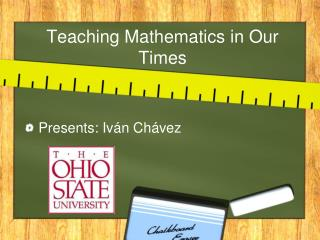DownloadDownload PresentationTeaching Mathematics in Our Times

# Teaching Mathematics in Our Times

Download Presentation## Teaching Mathematics in Our Times

- - - - - - - - - - - - - - - - - - - - - - - - - - - E N D - - - - - - - - - - - - - - - - - - - - - - - - - - -
##### Presentation Transcript

1. Teaching Mathematics in Our Times Presents: Iván Chávez

2. Introduction • Mathematics is a very powerful tool in multiple activities from solving an equation in one variable or to decode a complex code. • The teaching in general and of the mathematics particularly are matters of greater importance for the contemporary society.

3. Teaching mathematics in the past • Teaching Mathematics in 1800aC. • Teaching Mathematics in Mesopotamia • Teaching Mathematics in Mexico

4. This intense social process or scientific acculturation, has helped us to recognize the educational need to implement modifications in the field of the mathematics based on better designs adapted the scholastic practices.

5. Teach Mathematics is based in Mathematical Didactics Mathematical Logic

6. Mathematical Didactics • The tools to teach in several topic • Classics

7. Using our hands for multiply • Pay attention!

8. Popular, but little used

9. Accessible

10. Using a computer • If we want to know the stability of Differential System Equations at (0,0) • x´= x^2 +y^3 • y´= y^2 • If we choose Hartman-Grobman theorem we get the null matrix . • Using isoclines and nullclines (Program)

11. Mathematical Logic • The way to understand a problem and give the solution. • Have a lot of intuition

12. Understanding the limit idea • This is an example where you mix geometric and logical intuition

13. Understanding the Euclidean Geometry

14. No desperate, and be carefully when we have a problem.

15. For example other way to solve the Differential Equation System is with Lyapunov Stability. • This is a problem, because there isn't a way to choose a Lyapunov function, there are theorems but them are with specific conditions. • Perhaps we think in a circle, because meets the Lyapunov conditions, this is a idea.

16. Find a good method of study • Mnemotecnia • “Chicharronera” Formula • Song (Go to http://www.youtube.com/watch?v=HRcj9slciqM) • “x equals opposite bplus or minus the square rootb square minus 4acdivided by 2a”.

17. Trigonometric function • “Borracho” (Boozy) Formula in Mexico • Sohcahtoa in Puerto Rico

18. Integration by parts in Spanish “UnaVacaVestida de Uniforme”

19. Making funny stories • Solving a lot of problems

20. Find on Internet • We need to promote these resources

21. Mix of logical and didactical mathematical • Teaching mathematics always is new, because every day is a new way to understand and they are revolutionizing mathematics education. • Teaching mathematics are recognized by the scientific community , because in conjunct, do a hard work to offer the best way to learn math Conclusion

22. REMEMBER, don't memorize, because our brain works associating ideas and mix all resources mentioned. • We should to take very seriously teaching mathematics, helping our students, using every resource that we have, establishing an ambit of reliable and kindness. • We have the FUTURE in our hands to have excellent students and then a productive country.

23. Dedicated to: • Professor: Aurel Stan, I learned a lot of things about teaching, he always is very patient with me.He is an awesome professor • Professor: Rodica Costin, for the interview about the master degree in teaching mathematics. • Professor Luis Casian, for give me the opportunity to participate in SAMMS 2013. • Professor Martha Alvarez, my academic tutor in Mexico, and always helps me, with her advices and suggestions.